# What Does Modern Physics Say About The Number Of Our Dimensions ? - Part 1

in StemSocial4 months ago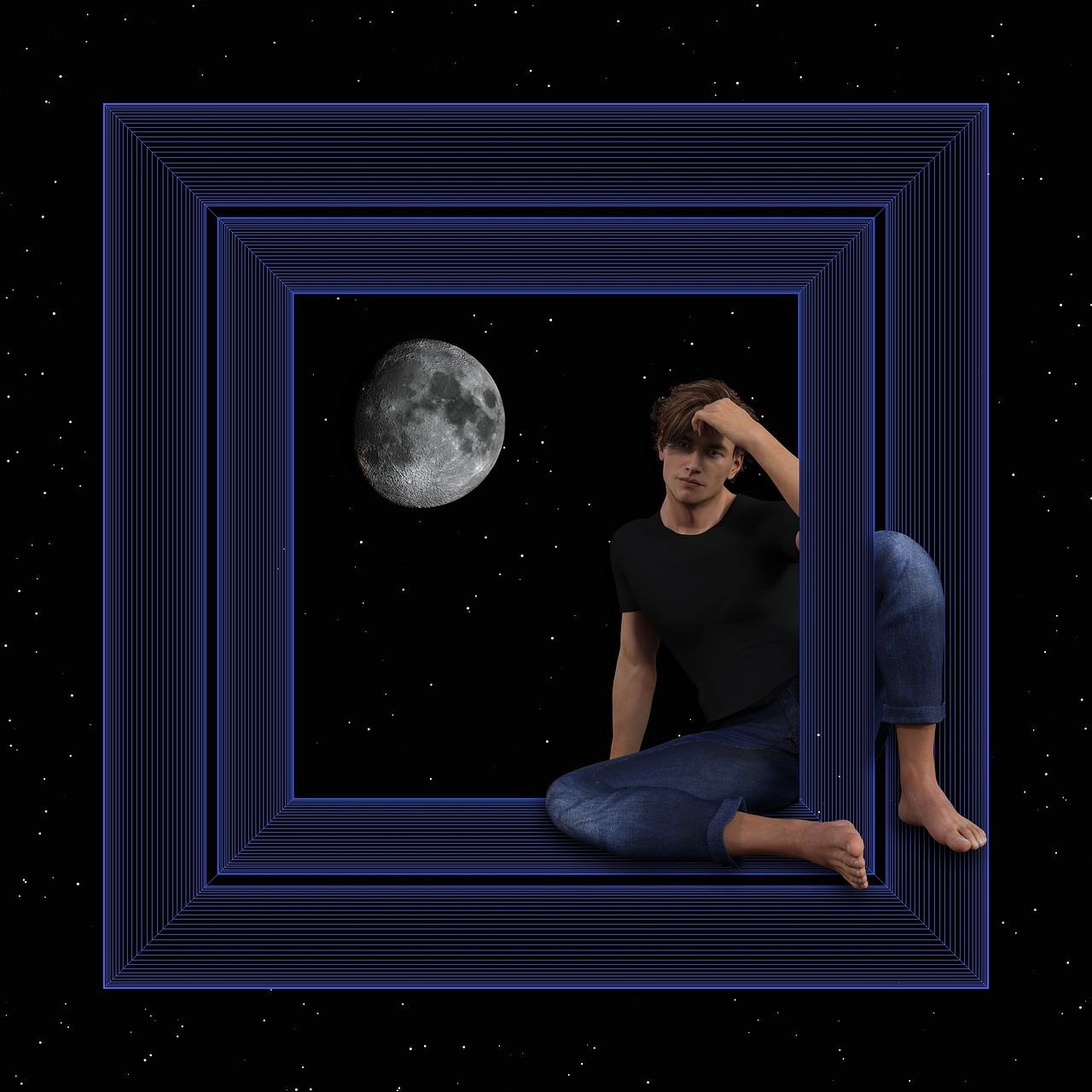Source

Modern Physics has a way of upending what you think you knew about our physical universe, especially if you are a layperson and as was seen in our previous article. Thanks to the inception of Einsteinian relativity and quantum physics, the universe now seems weirder than you can imagine. An aspect of Einsteinian relativity - general relativity, accurately describes gravity (especially at galactic scale) and can also be considered a generalization of Newtonian mechanics at classical level, this theory attempts to explain gravitational observations by taking into serious consideration the geometric structure of our four-dimensional universe.

Wait a minute, did I just say we exist in a four-dimensional universe ?

Sorry to break your hearts folks, it wasn't a mistake, we do actually exist in a four-dimensional universe according to general relativity. As a matter of fact, a four-dimensional universe is among the fundamental postulates of general relativity.

In our everyday experience, every physical object is observed to be three-dimensional, that is, if you have an object such as a box, these dimensions are it's height, length and width. These dimensions are labeled as h, L and w in the image below.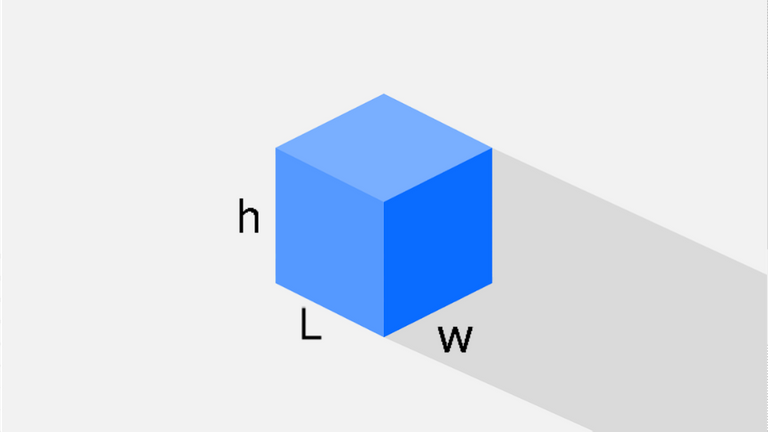Source

When objects move, they have many degrees of freedom and these degrees of freedom can be generalized into three spatial dimensions. When the object moves towards you at your front/back, that is one dimension and it can be labeled x, when the object moves towards you by your side, that is another dimension which can be labeled y and lastly, when the object moves towards you from above your head or below your feet, that is another dimension distinct from the other two (x and y), this third dimension can be labeled z. In each dimension, depending on the one the object is moving in, changes occurs (position changes) and these changes are fundamentally characterized with the concept of "time" and it's flow. This kind of physics is referred to as Newtonian mechanics, as time is not treated as a dimension like the other three (x, y and z) discussed earlier.

After the inception of Einsteinian relativity in the early 20th century, it was realized that time was in fact a dimension like the other three. According to this new physics, time is one of the two possible manifestations of a single entity called spacetime, the other manifestation being space, just like graphite (used in pencils) and diamonds (used in some jewelries) are different manifestations of the same chemical element "carbon". When objects move, according to Einsteinian relativity there always exists the time dimension (sometimes called the temporal dimension) in addition to the spatial dimensions (x, y and z).

When objects move freely, they always trace a geometric path (sometimes called a geodesic), for example, if an object moves freely along the surface of a ball, it's geodesic would be curved (an arc of a circle or a full circle), when the same object moves freely on the surface of a flat sheet of paper, it's geodesic is a straight line. From the Newtonian perspective and assuming the geodesic is a full circle, the position of the moving object (including it's past and future position) can be described with coordinates a and b that makes up/belongs to the spatial dimensions x and y. In Einsteinian relativity, there's always attached to x and y another dimension labeled t (time dimension), technically, this time dimension is normally given as the mathematical expression ct (c is the vacuum speed of light and t is time). This time dimension also contributes to the geometry of the object's motion . In the case of our object moving freely along the surface of the ball, the full geometry of it's motion/geodesic isn't a circle, it could be a sphere like the ball or a cylinder or any three-dimensional shape that possesses the curved spatial dimension, in coordinate terms, events in both space and time can be described with labels a, b and d which make up/belongs to the dimensions x, y and ct.

Physically, we only observe the shape/geometry of space of the moving object, which in the case of our object moving freely along the surface of a ball can be a full circle, we don't normally observe the complete shape of the object's motion - time dimension seems invisible, but time dilation which has been confirmed experimentally is an indication that time makes up the geometry. To know what the complete geometry/shape is, a correct mathematical model (using the geodesic equations of Einsteinian relativity) would be needed. In the real world, the full geometry of motion can be four-dimensional (including the time dimension) but we can only observe that of space (three-dimensional).

Higher than three dimensional objects are very difficult to visualize and it's because we are not used to it, there's no physical object that has spatial dimensions higher than three. If a higher than three dimensional object happens to exist in our world, it would still appear three-dimensional. An example is the case of an object called the "tesseract" - a four-dimensional cube, the image below shows how to construct a tesseract (number 4) from scratch (number 0 - a point).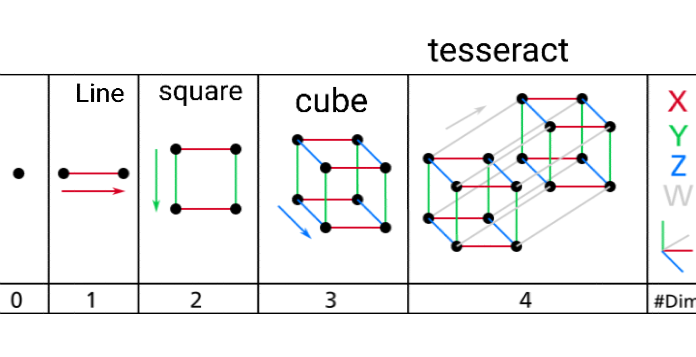Source

The actual image of the tesseract is given below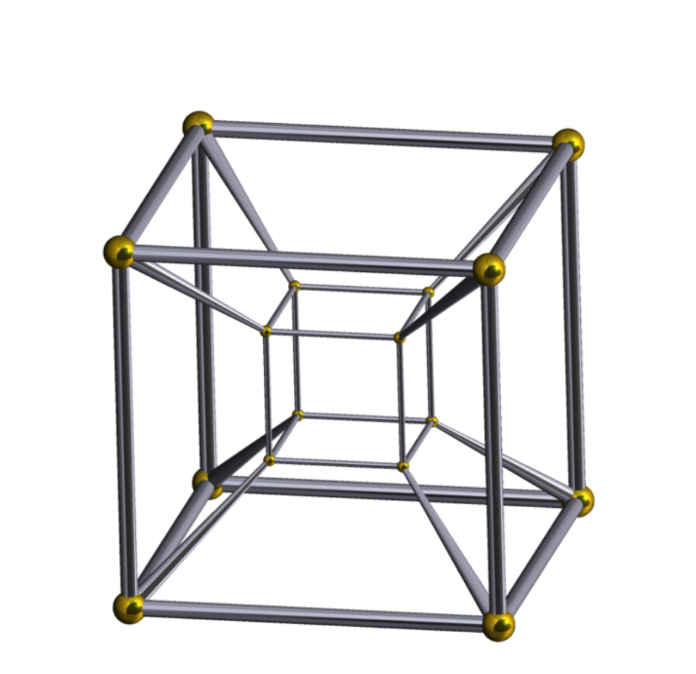Source

Notice that it looks like a big cube containing in it's inside a small cube (it still looks three-dimensional) but in the actual fact, both cubes are of the same size. The view above is what would be expected when observed in our world. The proper visualization of the tesseract is given below.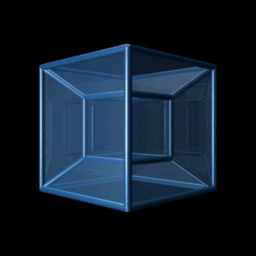Source

Notice that as we rotate the object, the cube that appears small on the inside eventually becomes the big cube and vice versa.

If we assume that the tesseract above is the full motion geometry/geodesic of spacetime in the absence of matter/energy, that is, no matter/energy in the tesseract, then according to general relativity, the introduction of matter/energy into the tesseract would deform/change the geometry, this deformation is normally in a curved manner. If the distribution of matter/energy (amount of matter/energy per unit space) is extremely concentrated and uniform, then the geometry of this spacetime can change from the tesseract to a hypersphere - a four-dimensional sphere. This change in geometry is only noticed by an observer outside this spacetime, for observers inside the spacetime, he/she thinks the geometry is still the tesseract and would attribute certain new observations (after the change) to the "force of gravity". Other objects free falling in a gravitational field (the perspective of observers inside the hypersphere) are actually objects following the new geometric path - hypersphere, that is prescribed by the distributed matter/energy. For example, our planet (Earth) orbits around the sun in an orbit that is approximately elliptical (neglecting precession and the geometry of time), locally we say that it's the force of gravity that's responsible for keeping our planet in orbit but in the actual sense, Earth is simply following the geometric path prescribed by the sun.

We should note that the tesseract and hypersphere used in this article to describe gravity is for simple illustration. Technically, the motion geometry of a four-dimensional spacetime whether in the presence/absence of matter/energy is much more complicated.

Remember that gravity is among the four fundamental forces of nature, the other three being the strong force, weak force and electromagnetic force. These non-gravitational forces are not described by general relativity but by quantum physics. One theoretical discovery that baffles physicists even to this day, is the number of dimensions of the universe that is required for a successful model that unifies the two theoretical frameworks (general relativity and quantum physics) into a single theoretical framework or that is required to quantize gravity - describing gravity in the language of quantum physics. Believe me when i tell you, this is much more weirder and interesting than what general relativity tells us about the number of our dimensions, though these models are yet to be confirmed experimentally. This however would be a discussion for another time, probably in the next article following this one.

It's here we conclude this article, have a thoughtful day and see you next time.

Dimension

Spacetime

General relativity

Four-dimensional space

Thank you all once again for stopping by to read my jargons and also thank you @juecoree, @lemouth and the @Steemstem team for your valuable supports.Source

Lastly, please don't forget to do the needful
Upvote
Comment
Reblog
If you enjoyed my jargons.Sort:

Congratulations @clinton19! You have completed the following achievement on the Hive blockchain and have been rewarded with new badge(s):You received more than 10000 upvotes.Your next target is to reach 15000 upvotes.

If you no longer want to receive notifications, reply to this comment with the word `STOP`

Check out the last post from @hivebuzz: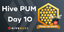Hive Power Up Month - Feedback from day 10
###### Support the HiveBuzz project. Vote for our proposal!Thanks for your contribution to the STEMsocial community. Feel free to join us on discord to get to know the rest of us!

Please consider delegating to the @stemsocial account (85% of the curation rewards are returned).

You may also include @stemsocial as a beneficiary of the rewards of this post to get a stronger support.

4 months ago (edited)

Nice piece of text, very well written. Thanks for sharing it with us!

Note that I enjoyed in particular the illustration of the tesseract :)

Note that I enjoyed in particular the illustration of the tesseract :)